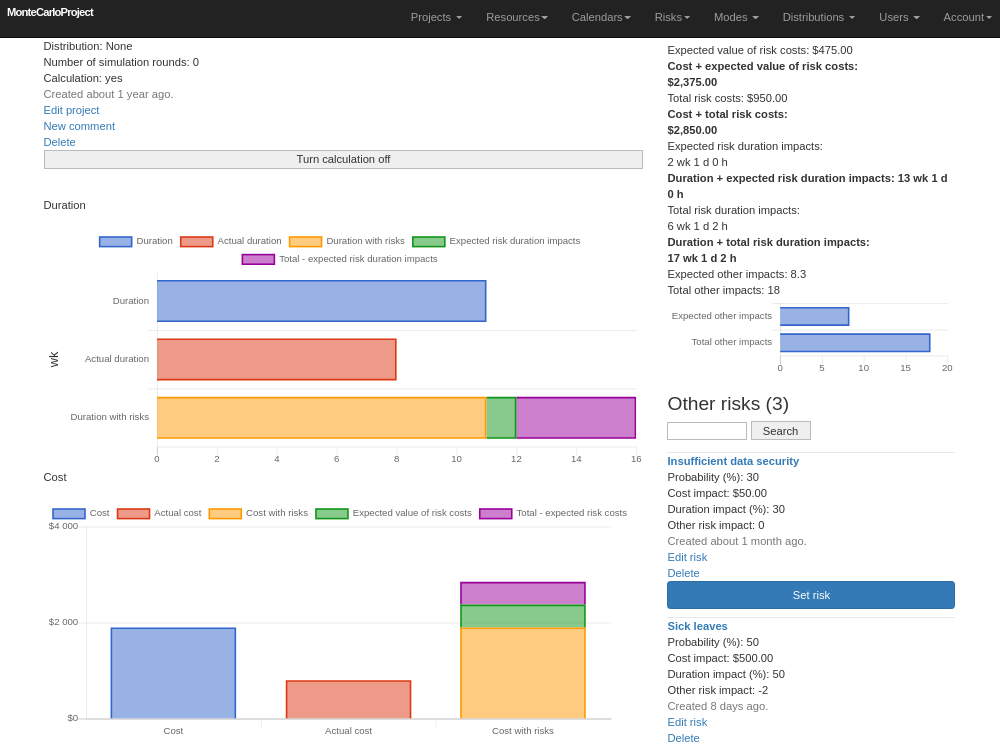Risk management is a new feature in MonteCarloProject.

Risk management requires MonteCrystal plan.

A company or organization can describe different risks that can be attached into projects or into their tasks. Name, description and the following other information can be given for each risk:

• Probability of occurrence (%)
• Cost impact type (absolute or relative). Absolute impact is added as such to the planned cost of a task or a project. Relative impact is calculated based on the planned cost. For example a risk having a 30% relative impact will increase the task's cost by \$300 if its planned cost is \$1000.
• Cost impact given as absolute (\$) or relative (%) amount depending on the chosen cost impact type
• Duration impact (%)
• Other risk impact on a scale of -10 ... +10. Negative numbers reflect negative risks, i.e. an impact where performance is better than planned.

A risk calculation rule can be set for project tasks. Rule determines how risk affected cost and duration are calculated for the task. Following options are available:

• 'Risks not calculated'. Risks do not affect task cost or duration.
• 'Expected value of risks'. Expected values of assigned risks' cost and duration are added to planned cost and duration.
• 'All risks'. Assigned risks' cost and duration impacts are added as such to planned cost and duration. Probabilities are not considered.

For the assigned risks their impact on task or project cost, duration and other factors (for example resulting benefits) is calculated. Both expected value and total impact is shown (picture 1).Picture 1. Risk impacts on the project.

The calculated risk impacts can be further utilized in the project simulation.

Save